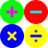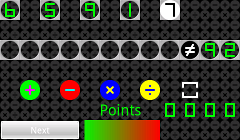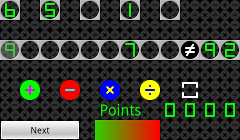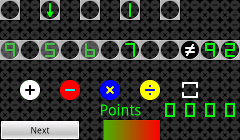Sumudo

Sumudo is a game of mathematical dexterity and imagination. The aim of the game is to create mathematical expressions which equates to the randomly generate result, by placing the randomly generated single digit numbers into the slots and join them up with mathematical symbols, thereby creating equations with the specified result. You have a limited amount of time to do this in.

To start, select a number by tapping it. It changes to a white circle with the number in to show that you have selected it.Now, select the slot you want the number to go in by tapping the slot in the equation line. The number slots in the equation line are those below the numbers in the number line.When you have placed a second number, you may noticed that one of the numbers is greyed out.Equations are evaluated by taking the first valid equation nearest the equals sign. So, in this instance, only the 7 is evaluted.

Note that, if you select a number which is already in the equation, it reverts to its starting position, and remains selected. To unselect the number, just tap anywhere away from the equation line.

If a number is place directly below its starting position, it will carry a bonus value when it comes to scoring (see later), and this is indicated by an arrow pointing downwards.To add a mathematical symbol to the equation, select a symbol by tapping it. It changes to a white circle with the symbol in to show that you have selected it.Now, select the slot you want the symbol to go in by tapping the slot in the equation line. The symbol slots in the equation line are those above the symbols in the symbol line.Note that, the symbol is not moved to the equation line, just copied, and that you can replace a symbol in the equation by just copying another one on top of it.

When determining the result of the equation, standard operator precedence is used. This means that multiplications and divisions are calculated before additions and subtractions. For example, 9x5+6-7 equates to 44 (9 is multiplied by 5 before it is added to 6, then 7 is subtracted), not 92 (where 5 is added to 6 before being multiplied by 9, then subtracting 7).This can be overridden by the use of brackets. Brackets can be applied by selecting the bracket operation. It changes to a white square with the symbol in to show that you have selected it.Now, select the mathematical operation in the equation to be bracketed.If there is more than one operation to be taken into account in the bracket, drag across the operations during your selection. Brackets can be removed by repeating the procedure. Brackets to be added will be shown in green, whereas brackets to be removed will be shown in red.Attempts to place brackets incorrectly, for example, overlapping brackets, are shown in yellow.The more digits you use, the higher your score. The first digit used is worth 5 points, the second digit used is worth 10 points, the third digit used is worth 15 points, and so on. If the digit is placed directly below its starting position (indicated by an arrow pointing downwards) there is a bonus of 5 points, making the maximum score 100 points. There is no score for an unequal equation.

When you create a valid equation, you can go on to the next set of numbers. You may also go on to the next set of numbers after 30 seconds. Since each set of numbers is generated randomly, it is possible that there is no solution, in which case, you will need to wait for 30 seconds before going on to the next set of numbers.

The full version can be played in three modes, a 1 minute game, a 3 minute game or a 5 minute game. At the end of the game, no more points can be added to your total score, although you will be able to keep trying to create a valid solution.There is also a bluetooth challenge version (Sumudo BT), where you can challenge someone to a match, their score being shown below yours. Both players need to have the BT version and have Android 2.0 or higher on a bluetooth enabled device.